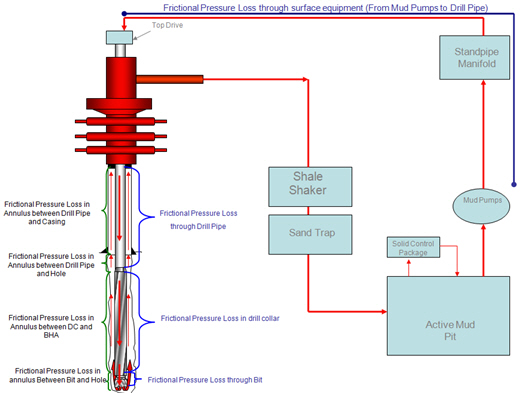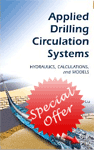# Total Pressure Loss through The Drilling System Without Bit

We’ve learned several calculations regarding pressure loss in the drilling system and today, we will combine all knowledge together so we will get the total pressure loss in the system excluding the bit.Without downhole tool, the total pressure loss without bit is equal to summation of pressure loss at the surface equipment, pressure loss through drill string (drillpipe and drill collar) and pressure loss in the annulus.

The equation below demonstrates the system pressure loss excluding the bit:Where;

Po = the system pressure loss excluding the bit

Cse = coefficient of surface equipment

Lp = length of drill pipe

Cpa = coefficient for annulus of the drill pipe

Cpb = coefficient through bore of drill pipe and tool joint

Lc = length of drill collar

Cca = coefficient for annulus of the drill collar

Ccb = coefficient through bore of drill collar

W = mud weight, ppg

PV = plastic viscosity of drilling mud, centipoise

Vf = viscosity correction factor

Q = flow rate, gpm

In the next topic, we will apply this concept to determine the optimum flow rate for the basic system.

Reference:Drilling Hydraulic Books

Share the joy1.Hugo Soriano Martínez says: